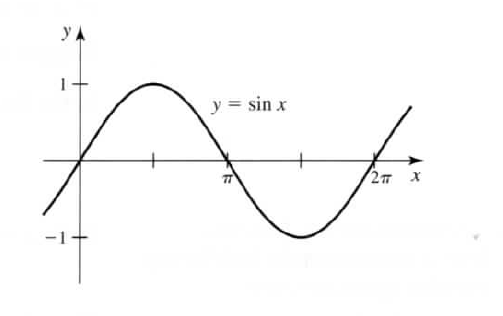×
Get Full Access to Calculus: Early Transcendentals - 1 Edition - Chapter 3.1 - Problem 72ae
Get Full Access to Calculus: Early Transcendentals - 1 Edition - Chapter 3.1 - Problem 72ae

×

# Graph of the derivative of the sine curve a. Use the grISBN: 9780321570567 2

## Solution for problem 72AE Chapter 3.1

Calculus: Early Transcendentals | 1st Edition

• Textbook Solutions
• 2901 Step-by-step solutions solved by professors and subject experts
• Get 24/7 help from StudySoup virtual teaching assistantsCalculus: Early Transcendentals | 1st Edition

4 5 1 282 Reviews
25
2
Problem 72AE

Graph of the derivative of the sine curve

a. Use the graph of y = sin x (see figure) to sketch the graph of the derivative of the sine function.

b. Based upon your graph in part (a), what function equals $$\frac{d}{dx}(\sin x)$$?Step-by-Step Solution:
Step 1 of 3

SOLUTION STEP 1 (a). We need to sketch the derivative of the given sine graph We know that f(x)is the slope of the tangent at the point (x,f(x)) on the graph of f. From this graph we can find that at points x = and at x = 3 the slope is 0. Thus the graph 2 2 of y’ intercepts the x axis at these points. Then we can find that the slope is positive(ie, increasing slope) from x = to x = and f2om x = the slope decreases(ie,negative) upto x = 3and then it again increases upto x = 2 2 2 .Thus the graph of the derivative will be This is the graph of a cosine function. STEP 2 (b).Thus we can see that the derivative of the sine function is cosine function Therefore dxsin x = cos x

Step 2 of 3

Step 3 of 3

##### ISBN: 9780321570567

The answer to “?Graph of the derivative of the sine curvea. Use the graph of y = sin x (see figure) to sketch the graph of the derivative of the sine function.b. Based upon your graph in part (a), what function equals $$\frac{d}{dx}(\sin x)$$?” is broken down into a number of easy to follow steps, and 41 words. This full solution covers the following key subjects: graph, function, Sine, derivative, aph. This expansive textbook survival guide covers 112 chapters, and 7700 solutions. Calculus: Early Transcendentals was written by and is associated to the ISBN: 9780321570567. Since the solution to 72AE from 3.1 chapter was answered, more than 460 students have viewed the full step-by-step answer. This textbook survival guide was created for the textbook: Calculus: Early Transcendentals, edition: 1. The full step-by-step solution to problem: 72AE from chapter: 3.1 was answered by , our top Calculus solution expert on 03/03/17, 03:45PM.

## Discover and learn what students are asking

Calculus: Early Transcendental Functions : Second-Order Nonhomogeneous Linear Equations
?Verifying a Solution In Exercises 1-4,verify the solution of the differential equation. Solution D

Statistics: Informed Decisions Using Data : Assessing Normality
?A ______ ______ ______is a graph that plots observed data versus normal scores

Unlock Textbook Solution